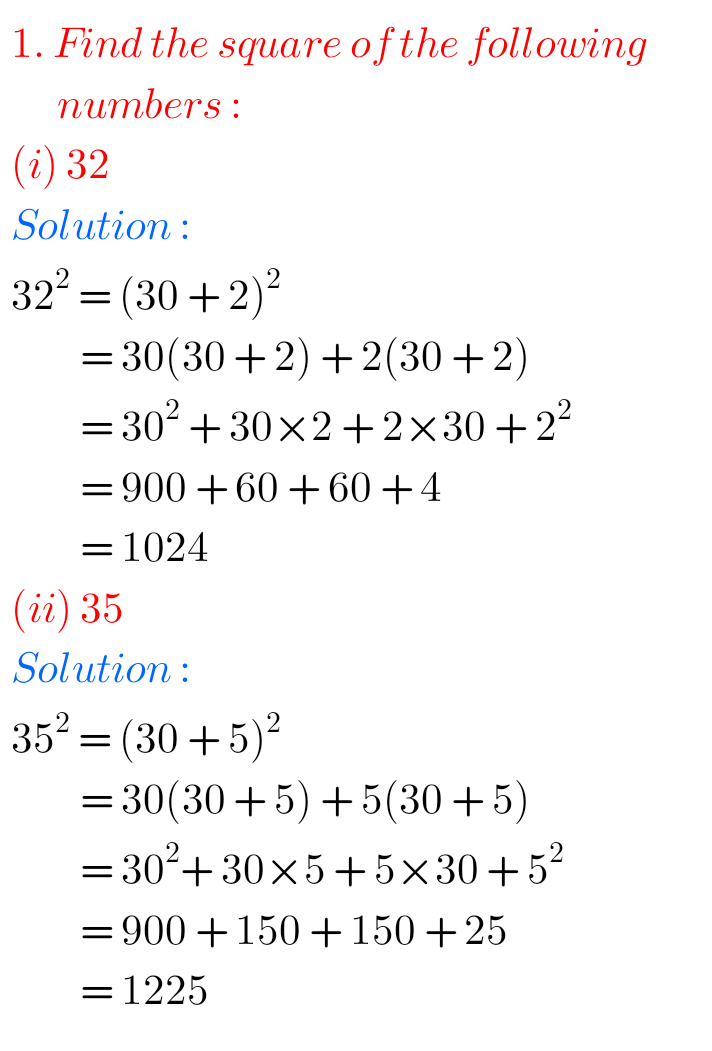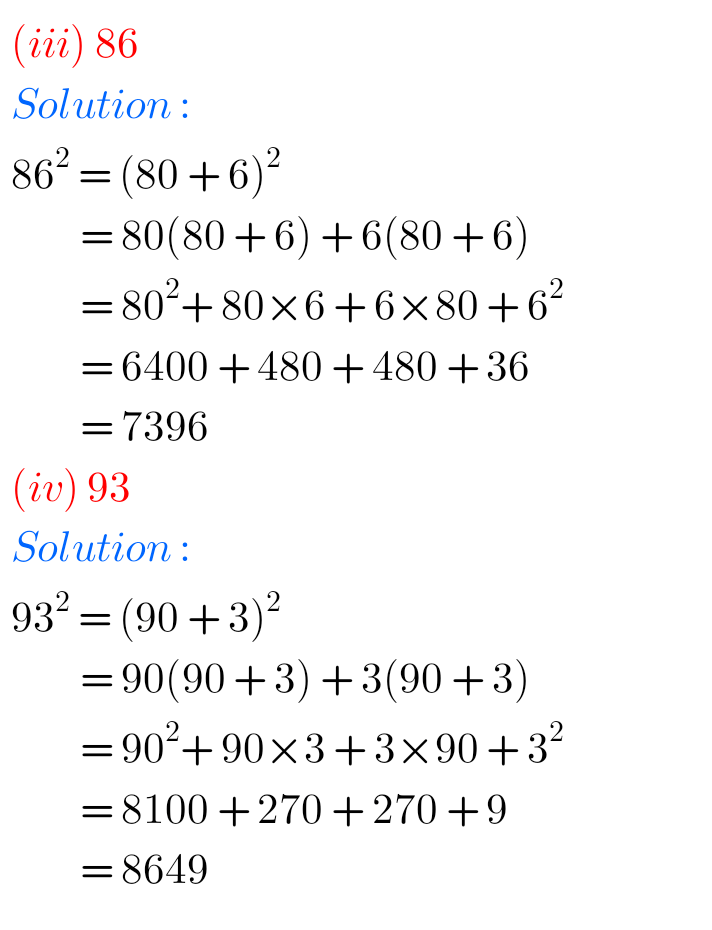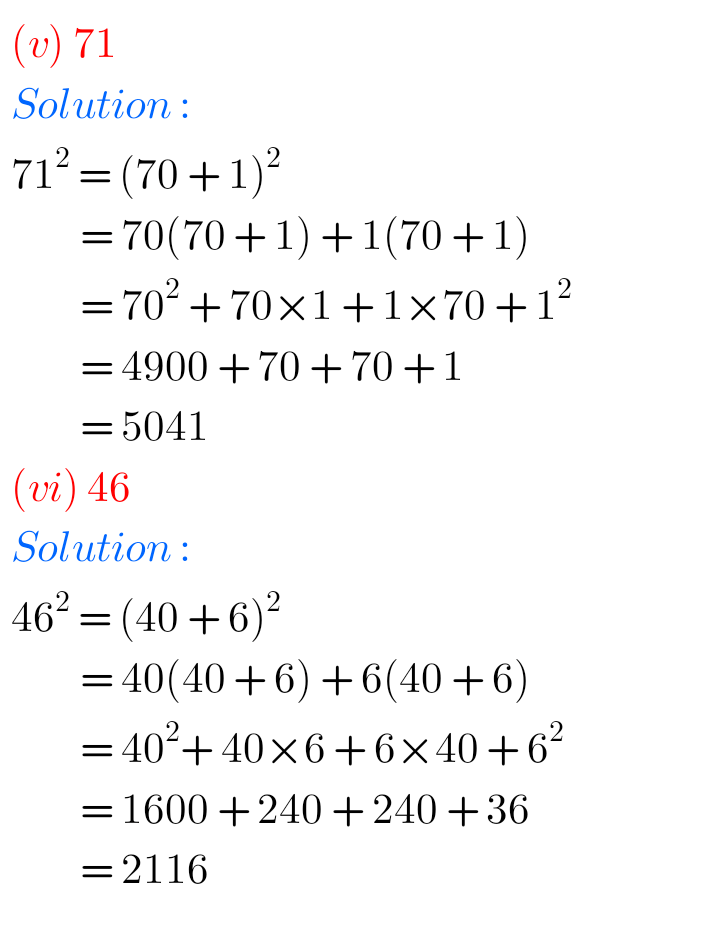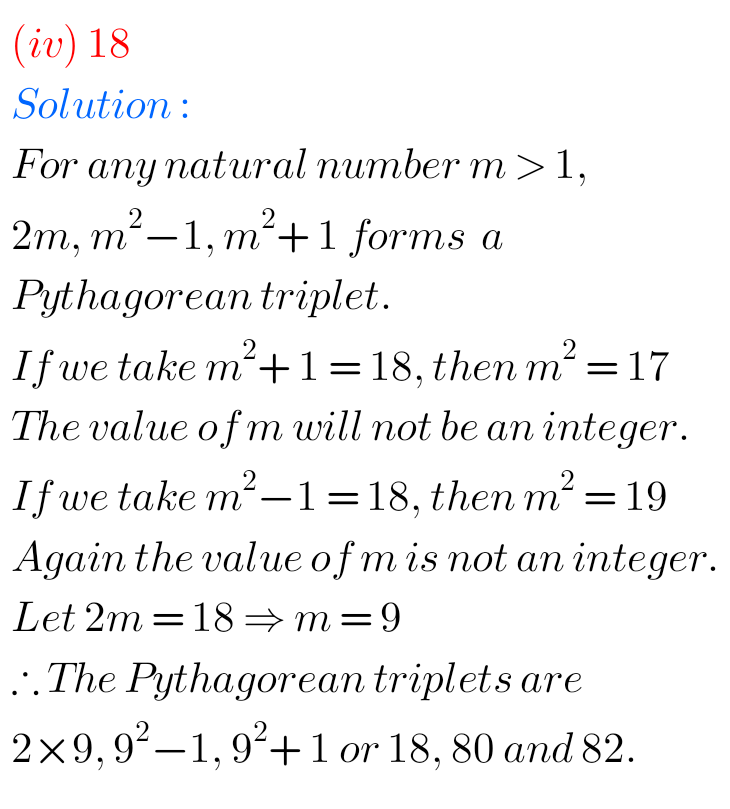# Squares and Square Roots exercise 5.2 solutions Class 8 NCERT

NCERT mathematics class 8 chapter 5 Squares and Square Roots exercise 5.2 solutions are given.

You should study the textbook lesson Squares and Square Roots very well.

You should also observe and practice all example problems are given in the textbook.

Observe the given solutions and try them in your own method.

Chapter 2 Linear Equations in one Variable

Squares and Square Roots

Exercise 5.1

Exercise 5.2

Exercise 5.3

## Maths solutions for Squares and Square Roots exercise 5.2 solutions NCERT

Chapter 5

Squares and Square Roots

Exercise 5.2### Solutions for chapter 5 Exercise 5.2 solutions NCERT class 8Note: Observe the solutions and try them in your own method.

NIOS Maths 311 book 2 Solutions for some chapters

NCERT class 6 maths solutions

NCERT class 7 maths solutions

Inter maths solutions

SSC maths Solutions class 10